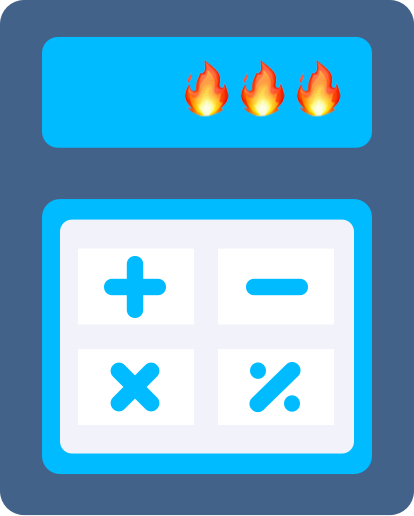Equal Fractions Calculator# Basic Fractions

#### Are fractions integers?

Great question! Fractions are not integers. Integers are whole numbers like 0 or 957 or negative numbers like -7. Think of a fraction like a piece of an integer, but not an integer itself.

But wait! You might have a fraction that simplifies into a whole number, like this:

Since 2 is an integer, and 2 is equal to , is also an integer. This is why it's helpful to always use the simplest form of the fraction.

#### Common Questions### What is a fraction?

If you've ever asked for "half" of anything, like half an apple or half a pitaya, you've used a fraction. A fraction is a number between whole numbers.

We represent a fraction using a number over a line over another number, like this:

The number on top (3) is called the numerator, and the number on the bottom (5) is the denominator. Remember it this way, denom kind of sounds like denim, and jeans are bottoms.### Can fractions be negative?

Fractions can be negative, just like any other number. In the same way that every integer has a negative and positive version of itself:

### 4

👇🏼
👇🏾

Fractions have a negative version on the other side of the number line, too. Try dragging one hand to see how the other matches up.

### 1

👇🏼
👇🏾

We show that a fraction is negative by putting a negative sign in front of it, like this:

But, if we have a negative sign on the top and bottom, the negatives actually cancel out, and our fraction is positive:

### Which fractions are equivalent to each other?

Let's say you're asked what fractions are equivalent to .

To answer this, we should remember that multiplying the top and bottom of a fraction by the same number doesn't change the value of the fraction. So,

This makes sense logically, because 3 is exactly half of 6, so .

That means there's an infinite number of fractions equal to any fraction you choose, because there's an infinite number of numbers we can multiply the top and bottom by!

### How do I know if two fractions are equivalent? Or which fraction is bigger?

Let's say you have these two fractions and you're trying to figure out if they're equal or if one is bigger than the other:

Right now, it's hard to compare, because the first fraction is out of halves and the second is out of fifths, like below.

What we need is for the total number of pieces to be the same for both, so that we can figure out which one is bigger or smaller. To do this, we multiply the top and bottom of each fraction by the denominator of the other fraction:

Now, because the first fraction is 5 out of 10 pieces and the second is 4 out of 10 pieces, we can clearly see the first fraction is bigger!

So, in our original fractions:

There you go! So, remember, when comparing fractions, first make sure the denominators are the same, so the size of the pieces on each side are the same.

### What does "undefined" mean?

If the denominator of our fraction is 0, then our fraction is undefined.

Normally, we can picture a fraction pretty easily. For example, is like having 1 of something, like a pizza, and 2 people to split it. Then, each person gets of a pizza 🍕.

But, if we have a 0 in the denominator like this:

Then, it's like saying there is 1 pizza and no one to split it. Trying to figure out how much each person gets when there are no people just doesn't compute.So, anytime we have a 0 in the denominator of a fraction, we can't do any Math with it, so we call it undefined.

If you need help with comparing two fractions, check out our greater than & less than lesson and calculator here!